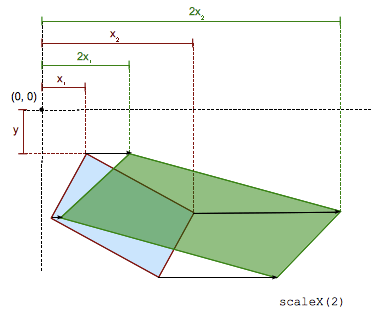/CSS

# scaleX()

The `scaleX()` CSS function defines a transformation that resizes an element along the x-axis (horizontally). Its result is a `<transform-function>` data type.It modifies the abscissa of each element point by a constant factor, except when the scale factor is 1, in which case the function is the identity transform. The scaling is not isotropic, and the angles of the element are not conserved. `scaleX(-1)` defines an axial symmetry, with a vertical axis passing through the origin (as specified by the `transform-origin` property).

Note: `scaleX(sx)` is equivalent to `scale(sx, 1)` or `scale3d(sx, 1, 1)`.

## Syntax

```scaleX(s)
```

### Values

`s`
Is a `<number>` representing the scaling factor to apply on the abscissa of each point of the element.
Cartesian coordinates on ℝ2 Homogeneous coordinates on ℝℙ2 Cartesian coordinates on ℝ3 Homogeneous coordinates on ℝℙ3
$\left(\begin{array}{cc}s& 0\\ 0& 1\end{array}\right)$ $\left(\begin{array}{cc}s0& 0\\ 01& 0\\ 0& 0& 1\end{array}\right)$ $\left(\begin{array}{cc}s0& 0\\ 01& 0\\ 0& 0& 1\end{array}\right)$ $\left(\begin{array}{ccc}s0& 0& 0\\ 01& 0& 0\\ 0& 0& 1& 0\\ 0& 0& 0& 1\end{array}\right)$
`[s 0 0 1 0 0]`

## Examples

### HTML

```<div>Normal</div>
<div class="scaled">Scaled</div>```

### CSS

```div {
width: 80px;
height: 80px;
background-color: skyblue;
}

.scaled {
transform: scaleX(0.6);
background-color: pink;
}
```